Next: Continuous Weights Mixture Model Up: Discrete Labels Mixture Model Previous: Non-spatial with Class Proportions

### Spatial

The prior on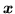is now a spatial prior. In this work we assume a discrete MRF (Besag, 1986; Geman and Geman, 1984). Taking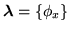, where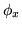is the MRF control parameter, which controls the amount of spatial regularisation. We have: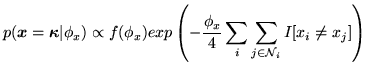(6)

whereis the spatial neighbourhood of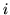(for this we use 26-connectivity in 3-dimensions),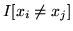is an indicator function (it is one if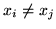and is zero otherwise), and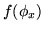is some unknown function of. This prior is identical to the prior used in Zhang et al. (2001); Salli et al. (1999), if the parameteris set to one. Usually,is hand tuned to work well for particular types of dataset. The best'' value forwill depend on the amount of, and topography of, the different classes. Marroquin et al. (2003) refer to this as the parameter which controls the granularity of the field, and they discuss how the use of different values for this parameter can affect the resulting segmented field. Indeed, we shall demonstrate later how fixing the amount of spatial regularisation to a single value will perform considerably less well than determining it adaptively from the data.

The hyperprior we use onis a non-informative Gamma distribution: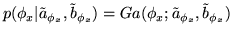(7)

Using all of this in equation 1, the posterior becomes: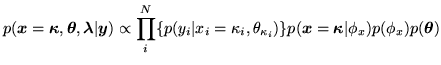(8)

Clearly, a fourth mixture model could be considered. This would be a spatial mixture model with global class proportions. However, it is far from clear how we would combine the prior on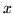in equation 4 with that in equation 6. Anyway, as we shall see in the results, we obtain good global histogram fits with the spatial mixture model specified here without including global class proportions.Next: Continuous Weights Mixture Model Up: Discrete Labels Mixture Model Previous: Non-spatial with Class Proportions